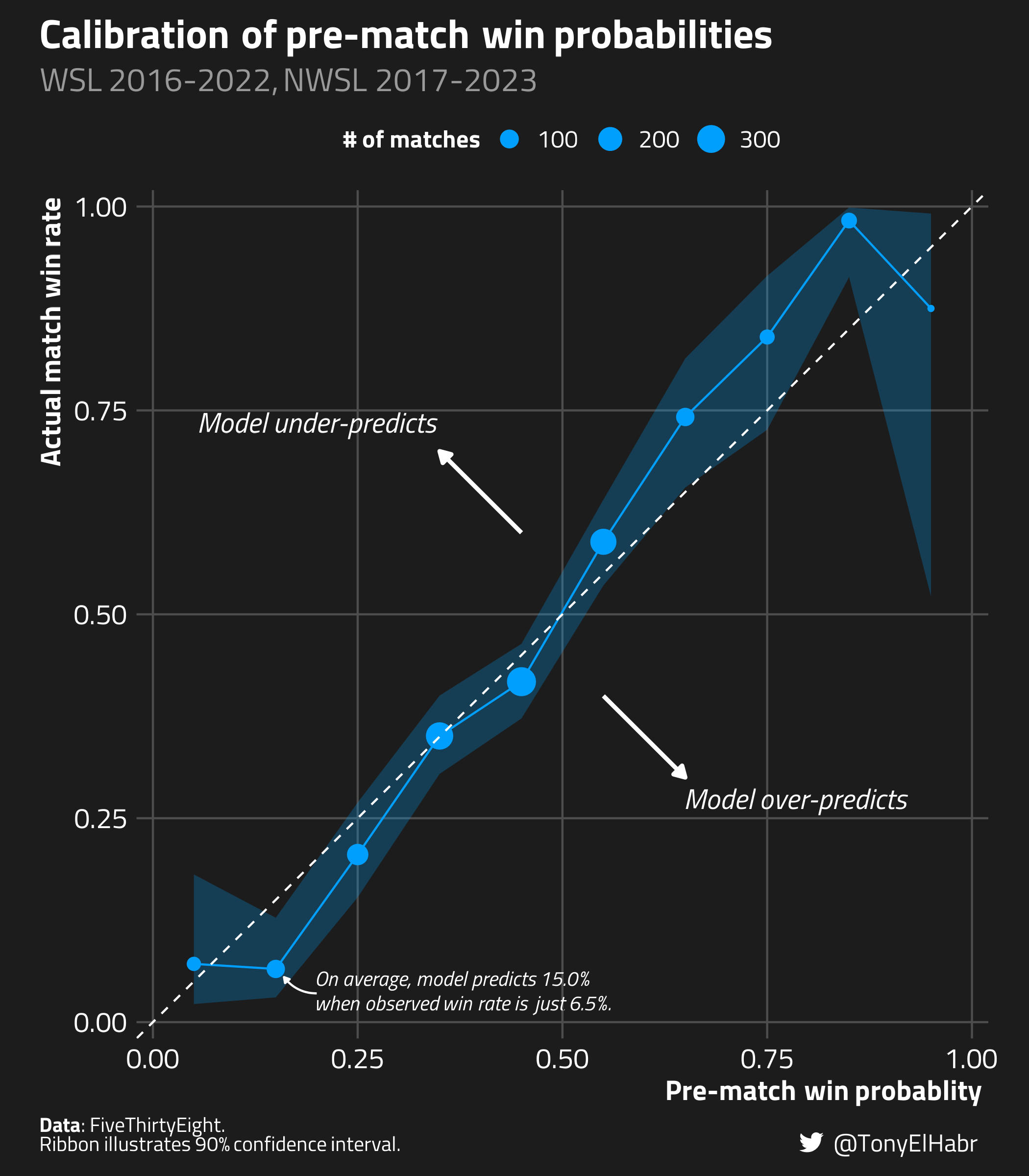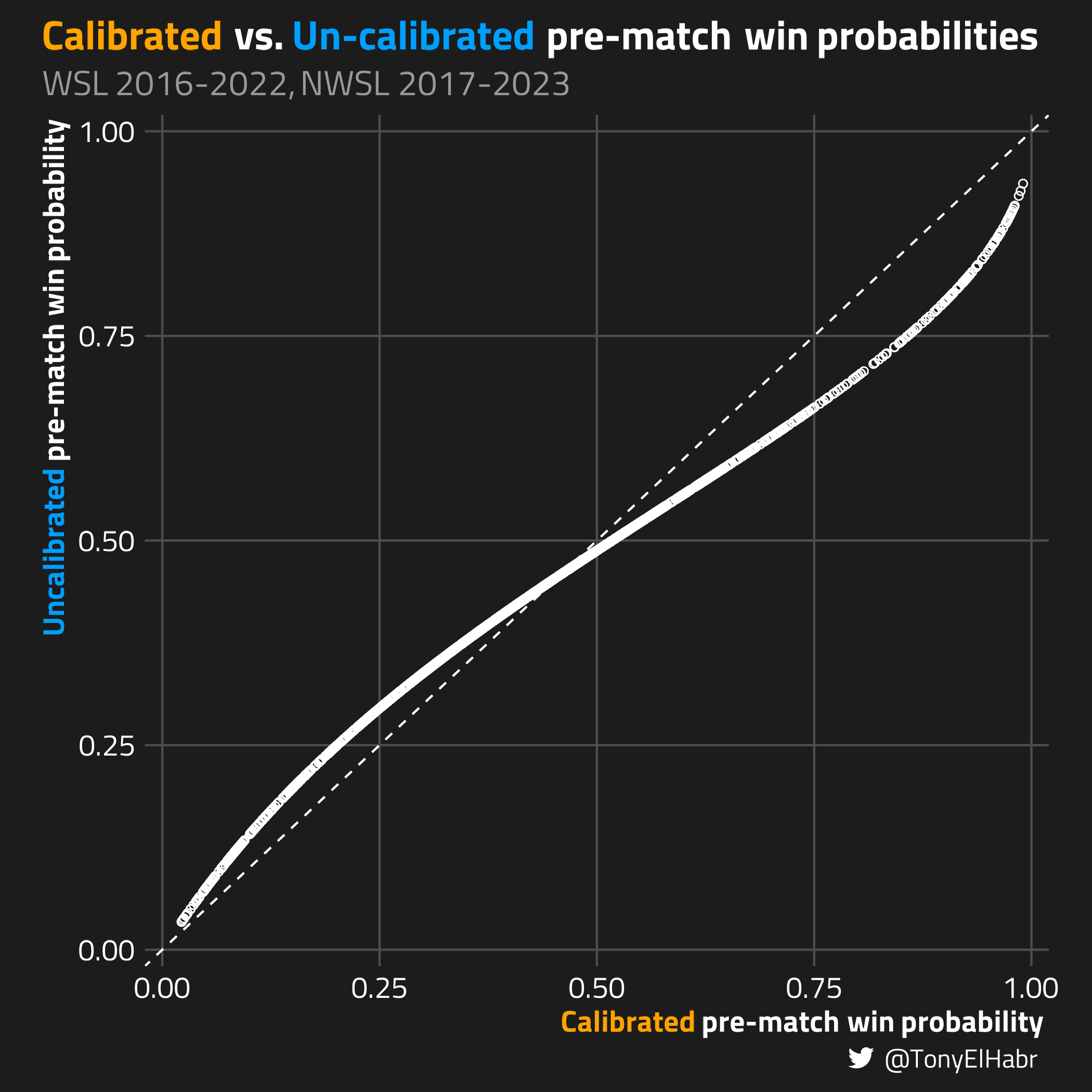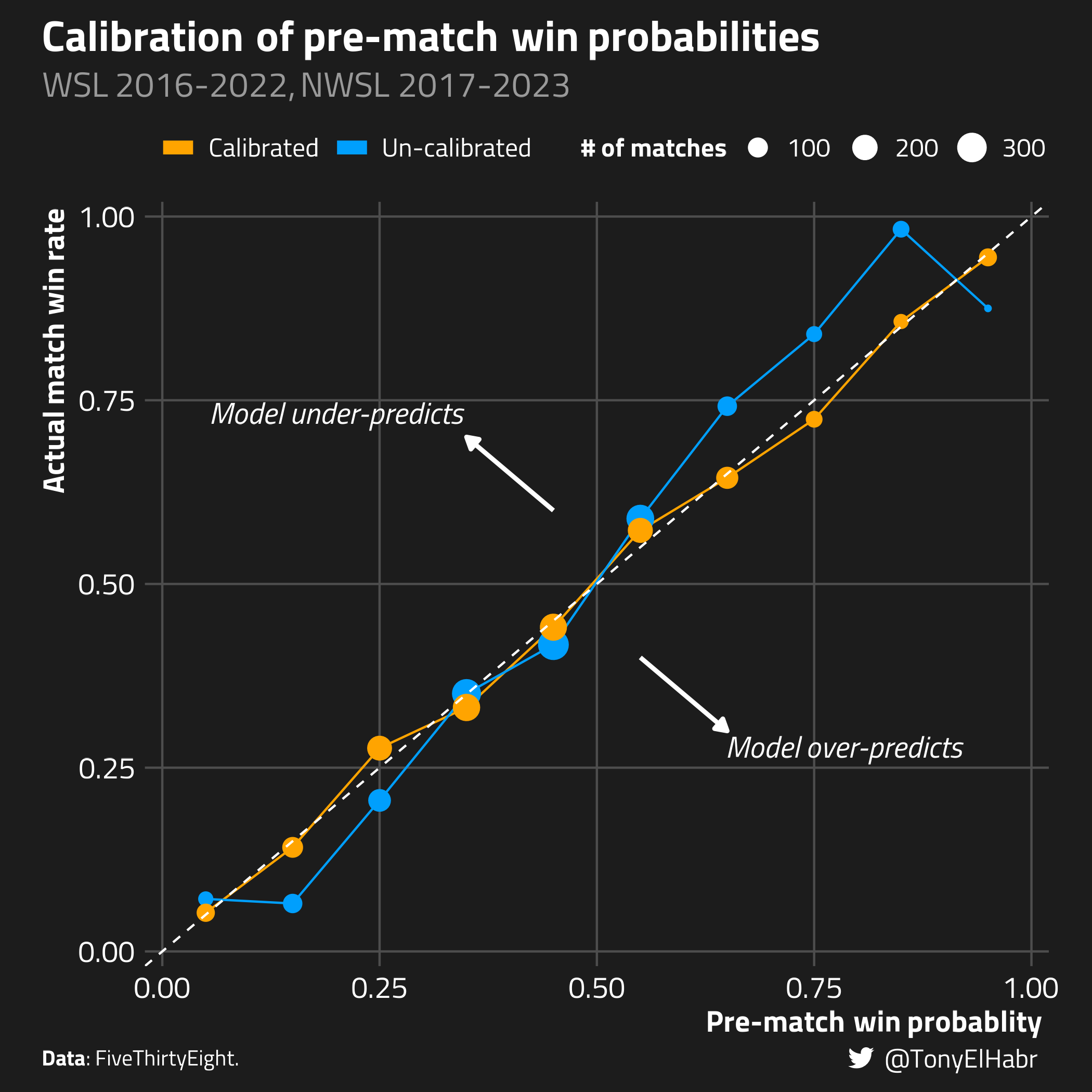# Calibrating Binary Probabilities

r
soccer
Using calibration to improve classifier model performance
Author

Tony ElHabr

Published

September 11, 2023

Modified

September 12, 2023

## Introduction

Ever grappled with a classification model that consistently over-predicts or under-predicts an event? Your first thought might be to re-evaluate the model’s features or framework. But what if tweaking the model isn’t an option, either due to a lack of resources or access restrictions? The good news is, there’s another way–it’s called calibration.

Calibration falls in the “post-processing” step of predictive modeling.1 We modify the output of a model using nothing but the model predictions and labels of the output. We do that by, you guess it, fitting another model, often called a “calibrator”.

• the pre-processing stage (e.g., feature engineering, normalization, etc.)
• model fitting (actually training the model)
• post-processing (such as optimizing a probability threshold)

One of my favorite (and relatively new) packages in the {tidymodels} ecosystem is the {probably} package. It provides functions that make it fairly straightforward to do calibration, even for those who are new to the concept. So let’s use {probably} to demonstrate the power of calibration.

## Calibrating a Binary Classifier

### Data

I’ll be using the pre-match win probabilities from FiveThirtyEight (RIP). Specifically, I’ll subset their plethora of historical projections to two women’s leagues: the Women’s Super League (WSL) in England and the National Women’s Soccer League (NWSL) in the U.S. I have a suspicion that the model probabilities are not as calibrated as they could be, as has been observed that gender-agnostic models in soccer can be less performant for the women’s game.

To keep things simple, I’m going to treat this as a binary classification task, where matches are the “positive” outcome ("yes"), and losses and draws are grouped as the “negative” outcome ("no").

Retrieve data
library(readr)
library(dplyr)
dplyr::filter(
!is.na(score1), ## match is completed
league %in% c(
"FA Women's Super League",
"National Women's Soccer League"
)
) |>
dplyr::transmute(
league,
season,
date,
team1,
team2,
target = factor(ifelse(score1 > score2, 'yes', 'no')),
## .pred_ is sort of a convention among {tidymodels} docs
.pred_yes = prob1,
.pred_no = 1- .pred_yes
)
matches
#> # A tibble: 1,358 × 6
#>    date       team1               team2             target .pred_yes .pred_no
#>    <date>     <chr>               <chr>             <fct>      <dbl>    <dbl>
#>  1 2016-07-09 Liverpool Women     Reading           yes        0.439    0.561
#>  2 2016-07-10 Arsenal Women       Notts County Lad… yes        0.357    0.643
#>  3 2016-07-10 Chelsea FC Women    Birmingham City   no         0.480    0.520
#>  4 2016-07-16 Liverpool Women     Notts County Lad… no         0.429    0.571
#>  5 2016-07-17 Chelsea FC Women    Arsenal Women     no         0.412    0.588
#>  6 2016-07-24 Reading             Birmingham City   no         0.382    0.618
#>  7 2016-07-24 Notts County Ladies Manchester City … no         0.308    0.692
#>  9 2016-07-31 Arsenal Women       Liverpool Women   no         0.435    0.565
#> 10 2016-08-03 Reading             Manchester City … no         0.306    0.694
#> # ℹ 1,348 more rows

### Diagnosis

We start with the “diagnosis” phase: “How well do the original probabilities perform?” To evaluate this, we can use one of the several probably::cal_plot_* functions. In this case, we’ll use cal_plot_breaks().2

This function neatly divides the range of predicted probabilities from zero to one into distinct bins. For each bin, it calculates the observed event rate using data that has probabilities falling within that bin’s range. Ideally, if our predictions are calibrated, the curve produced should match up with a straight diagonal line, i.e. a 45-degree slope passing through (0,0) and (1,1). As a bonus, the probably::cal_plot_* family of functions even provides confidence intervals about the calibration curve.

library(probably)
packageVersion('probably')
#>  ‘1.0.1.9000’

matches |>
probably::cal_plot_breaks(
truth = target,
## the "_yes" in .pred_yes must match one of the values in target
estimate = .pred_yes,
## because "yes" is the second event level ("no" is the first)
event_level = 'second'
)

{probably} offers some really neat automatic plotting of calibration curves. While I’d suggest giving them a try, I like to make my curves in a certain manner. In particular, instead of using a “rug”, I like showing sample sizes via points on the curve.Indeed, it looks like there is some room for improvement with the match probabilities. It seems that the FiveThirtyEight model over-predicts when the actual win rate is low, broadly below 20%; and, likewise, it tends to under-predict when the true win rate is really greater than 60%

### Remediation

Now we move on to the “remedation” step. That is, we fit a model, a “calibrator”, with the binary outcome as the target variable and the probability estimate as the lone input feature. {probably} offers several options with the cal_estimate_*() set of functions.

I’ve opted for Beta calibration, although logistic calibration would work fine here as well. Beta calibration is a little more flexible, and, consequently, can provide superior probability estimates, especially when the target distribution is skewed.3

matches |>
probably::cal_estimate_beta(
truth = target,
estimate = dplyr::starts_with('.pred'),
event_level = 'second'
)
#>  ── Probability Calibration
#>  Method: Beta calibration
#>  Type: Binary
#>  Source class: Data Frame
#>  Data points: 1,358
#>  Truth variable: target
#>  Estimate variables:
#>  .pred_no ==> no
#>  .pred_yes ==> yes

Under the hood, cal_estimate_beta() is doing something like this.

library(betacal)

betacal::beta_calibration(
p = matches$.pred_yes, y = matches$target == 'yes',
parameters = 'abm'
)

It’s almost shocking how simple the implementation is.

## Results

With the calibrator model in hand, let’s make a scatter plot of all the points in the data set, viewing how the model has adjusted the original probabilities.We observe that the calibrator has increased point estimates on the lower end of the spectrum and decreased estimates on the upper end of the spectrum. The calibration has seemingly fine-tuned under-predicting and over-predicting behavior from the original model.

To see the change that calibrator has made, we can re-make our calibration curve plot, adding the “calibrated” curve alongside the original “un-calibrated” curve.Visually, it’s evident that the remediation has improved the probability estimates. The calibrated curve more closely “hugs” the ideal 45 degree slope across the whole probability spectrum.

### Validation

To quantitatively describe the difference in calibration between the two models, we can compare the Brier Skill Score (BSS) of the un-calibrated and calibrated models.4 Keep in mind that a higher BSS indicates a more calibrated model. (1 is ideal. 0 indicates that the model is no better or worse than a reference model5.)

Brier Skill Score (BSS) calculation
library(yardstick)
library(rlang)

brier_skill_score <- function(data, ...) {
UseMethod('brier_skill_score')
}

brier_skill_score <- yardstick::new_prob_metric(
brier_skill_score,
direction = 'maximize'
)

bss <- function(
truth,
estimate,
ref_estimate,
event_level,
case_weights,
...
) {

if (length(estimate) == 1) {
estimate <- rep(estimate, length(truth))
}

if (length(ref_estimate) == 1) {
ref_estimate <- rep(ref_estimate, length(truth))
}

estimate_brier_score <- brier_class_vec(
truth = truth,
estimate = estimate,
event_level = event_level,
case_weights = case_weights,
...
)

ref_brier_score <- brier_class_vec(
truth = truth,
estimate = ref_estimate,
event_level = event_level,
case_weights = case_weights,
...
)

1 - (estimate_brier_score / ref_brier_score)
}

brier_skill_score_estimator_impl <- function(
truth,
estimate,
ref_estimate,
event_level,
case_weights
) {
bss(
truth = truth,
estimate = estimate,
ref_estimate = ref_estimate,
event_level = event_level,
case_weights = case_weights
)
}

brier_skill_score_vec <- function(
truth,
estimate,
ref_estimate,
na_rm = TRUE,
event_level = yardstick:::yardstick_event_level(),
case_weights = NULL,
...
) {

yardstick:::abort_if_class_pred(truth)

estimator <- yardstick::finalize_estimator(
truth,
metric_class = 'brier_skill_score'
)

yardstick::check_prob_metric(truth, estimate, case_weights, estimator)

if (na_rm) {
result <- yardstick::yardstick_remove_missing(truth, estimate, case_weights)

truth <- result$truth estimate <- result$estimate
case_weights <- result$case_weights } else if (yardstick::yardstick_any_missing(truth, estimate, case_weights)) { return(NA_real_) } brier_skill_score_estimator_impl( truth = truth, estimate = estimate, ref_estimate = ref_estimate, event_level = event_level, case_weights = case_weights ) } brier_skill_score.data.frame <- function( data, truth, ..., na_rm = TRUE, event_level = yardstick:::yardstick_event_level(), case_weights = NULL ) { yardstick::prob_metric_summarizer( name = 'brier_skill_score', fn = brier_skill_score_vec, data = data, truth = !!rlang::enquo(truth), ..., na_rm = na_rm, event_level = event_level, case_weights = !!rlang::enquo(case_weights), fn_options = list( ref_estimate = ref_estimate ) ) } ## The BSS is more intuitive to caluclate using the match non-win rate. ## Accordingly, we'll use .pred_no for our probability. REF_ESTIMATE <- matches |> dplyr::count(target) |> dplyr::mutate(prop = n / sum(n)) |> dplyr::filter(target != 'yes') |> dplyr::pull(prop) raw_brier_skill_score <- brier_skill_score_vec( truth = calibrated$target,
estimate = calibrated$.raw_pred_no, ref_estimate = REF_ESTIMATE ) calibrated_brier_skill_score <- brier_skill_score_vec( truth = calibrated$target,
estimate = calibrated\$.pred_no,
ref_estimate = REF_ESTIMATE
)

compared_brier_skill_scores <- round(
c(
'Un-calibrated' = raw_brier_skill_score,
'Calibrated' = calibrated_brier_skill_score
),
3
)
compared_brier_skill_scores
#> Un-calibrated    Calibrated
#>         0.196         0.205

Indeed, we have (marginally) improved the original pre-match win probabilities. But this approach is arguably a little naive–we’ve only re-assessed the entire data set a single time without accounting for potential uncertainties.

Fortunately, the {probably} package provides the cal_validate_*() family of functions. These functions allow for a more rigorous assessment of whether the calibration enhances the original probabilities. We can generate resamples from the original data and then compute the average and standard error for our chosen metrics. This lets us compare the calibrated and uncalibrated probabilities more effectively.

Let’s do just that, using cross-validation with 10 folds and 10 repeats We’ll again use BSS to evaluate the model probabilities.

Robustly evaluate=ing calibrator
set.seed(42)
sets <- rsample::vfold_cv(
matches,
v = 10,
repeats = 10
)

## "fixed" in the sense that we're pre-defining the reference estimate.
##   I'm not sure there's another way of going about this when working in conjunction yardstick::metric_set() and probably::cal_validate_*()
##   with
fixed_brier_skill_score <- function(...) {
brier_skill_score(
ref_estimate = REF_ESTIMATE,
...
)
}

fixed_brier_skill_score <- function(data, ...) {
UseMethod('brier_skill_score')
}

fixed_brier_skill_score <- yardstick::new_prob_metric(
fixed_brier_skill_score,
direction = 'maximize'
)

eval_metrics <- yardstick::metric_set(
fixed_brier_skill_score
)

validation <- probably::cal_validate_beta(
sets,
truth = target,
metrics = eval_metrics
)

validation_metrics <- validation |>
tune::collect_metrics() |>
## i think the .config column is bugged (it just says "config" for all rows?)
dplyr::select(-dplyr::any_of('.config'))
validation_metrics
validation_metrics
#> # A tibble: 2 × 6
#>   .metric           .type        .estimator  mean     n std_err
#>   <chr>             <chr>        <chr>      <dbl> <int>   <dbl>
#> 1 brier_skill_score uncalibrated binary     0.196   100 0.00494
#> 2 brier_skill_score calibrated   binary     0.202   100 0.00628

We find that the calibrator does indeed offer a “significant” improvement when assessed through this more statistically rigorous method. Specifically, the mean BSS of the validation set, minus one standard error, exceeds the mean of the uncalibrated BSS by more than one standard error.

pivoted_validation_metrics <- validation_metrics |>
dplyr::transmute(
.type,
mean,
lower = mean - std_err,
upper = mean + std_err
)
pivoted_validation_metrics
#> # A tibble: 2 × 4
#>   .type         mean lower upper
#>   <chr>        <dbl> <dbl> <dbl>
#> 1 uncalibrated 0.196 0.191 0.201
#> 2 calibrated   0.202 0.196 0.209

## Conclusion

While I wouldn’t say “calibration is all you need”, it’s certainly something nice to have in your toolkit when you’re working on a modeling task. It can be a game-changer, especially when tweaking the original model isn’t an option, whether due to access limitations or, let’s be honest, sheer laziness.

Oh, and I failed to mention this earlier—calibration isn’t just for binary classifiers. Multinomial classifiers and even regression models can benefit from this technique as well.

Happy modeling, folks.

## Footnotes

1. The {tidymodels} guide breaks down the traditional modeling workflow into three steps:↩︎

2. Sticking with the basics, I use the defaults for num_breaks (10) and conf_level` (0.9).↩︎

3. We don’t have a skew problem in this context, but it’s good to note when a Beta calibration might provide meaningful benefits over other calibration methods.↩︎

4. In this case, I choose to use the observed match win rate as the reference model.↩︎